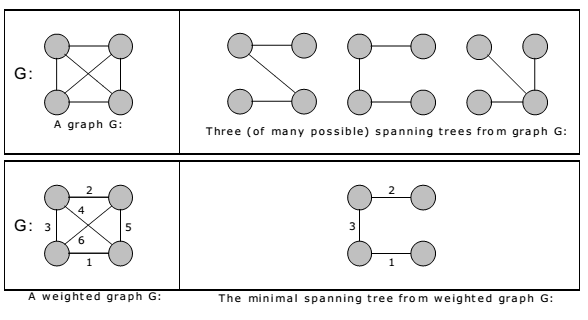# Minimum Spanning Tree (MST) A+ A  A-

A spanning tree for a connected graph is a tree whose vertex set is the same as the vertex set of the given graph, and whose edge set is a subset of the edge set of the given graph. i.e., any connected graph will have a spanning tree.

Weight of a spanning tree w(T) is the sum of weights of all edges in T. Minimum spanning tree (MST) is a spanning tree with the smallest possible weight.

Example:Let’s consider a couple of real-world examples on minimum spanning tree:

• One practical application of a MST would be in the design of a network. For instance, a group of individuals, who are separated by varying distances, wish to be connected together in a telephone network. Although MST cannot do anything about the distance from one connection to another, it can be used to determine the least cost paths with no cycles in this network, thereby connecting everyone at a minimum cost.
• Another useful application of MST would be finding airline routes. The vertices of the graph would represent cities, and the edges would represent routes between the cities. MST can be applied to optimize airline routes by finding the least costly paths with no cycles.

Minimum spanning tree, can be constructed using any of the following two algorithms:

1. Kruskal’s algorithm and
2. Prim’s algorithm.

Both algorithms differ in their methodology, but both eventually end up with the MST. Kruskal’s algorithm uses edges, and Prim’s algorithm uses vertex connections in determining the MST. In Prim’s algorithm at any instance of output it represents tree whereas in Kruskal’s algorithm at any instance of output it may represent tree or not.